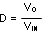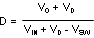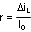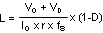SNVS288K September   2004  – September 2018

PRODUCTION DATA.

1. Features
2. Applications
3. Description
1.     Device Images
4. Revision History
5. Pin Configuration and Functions
6. Specifications
7. Detailed Description
1. 7.1 Overview
2. 7.2 Functional Block Diagram
3. 7.3 Feature Description
4. 7.4 Device Functional Modes
8. Application and Implementation
1. 8.1 Application Information
2. 8.2 Typical Applications
1. 8.2.1  LM2734X (1.6 MHz) VBOOST Derived from VIN 5V to 1.5 V/1 A
1. 8.2.1.1 Design Requirements
2. 8.2.1.2 Detailed Design Procedure
3. 8.2.1.3 Application Curves
2. 8.2.2  LM2734X (1.6 MHz) VBOOST Derived from VOUT 12 V to 3.3 V /1 A
3. 8.2.3  LM2734X (1.6 MHz) VBOOST Derived from VSHUNT 18 V to 1.5 V /1 A
4. 8.2.4  LM2734X (1.6 MHz) VBOOST Derived from Series Zener Diode (VIN) 15 V to 1.5 V / 1 A
5. 8.2.5  LM2734X (1.6 MHz) VBOOST Derived from Series Zener Diode (VOUT) 15 V to 9 V /1 A
6. 8.2.6  LM2734Y (550 kHz) VBOOST Derived from VIN 5 V to 1.5 V / 1 A
7. 8.2.7  LM2734Y (550 kHz) VBOOST Derived from VOUT 12 V to 3.3 V / 1 A
8. 8.2.8  LM2734Y (550 kHz) VBOOST Derived from VSHUNT 18 V to 1.5 V / 1 A
9. 8.2.9  LM2734Y (550 kHz) VBOOST Derived from Series Zener Diode (VIN) 15 V to 1.5 V / 1 A
10. 8.2.10 LM2734Y (550 kHz) VBOOST Derived from Series Zener Diode (VOUT) 15 V to 9 V / 1 A
9. Power Supply Recommendations
10. 10Layout
11. 11Device and Documentation Support
12. 12Mechanical, Packaging, and Orderable Information

• DDC|6

#### 8.2.1.2.2 Inductor Selection

The Duty Cycle (D) can be approximated quickly using the ratio of output voltage (VO) to input voltage (VIN):

Equation 14.The catch diode (D1) forward voltage drop and the voltage drop across the internal NMOS must be included to calculate a more accurate duty cycle. Calculate D by using the following formula:

Equation 15.VSW can be approximated by:

Equation 16. VSW = IO x RDS(ON)

The diode forward drop (VD) can range from 0.3 V to 0.7 V depending on the quality of the diode. The lower VD is, the higher the operating efficiency of the converter.

The inductor value determines the output ripple current. Lower inductor values decrease the size of the inductor, but increase the output ripple current. An increase in the inductor value will decrease the output ripple current. The ratio of ripple current (ΔiL) to output current (IO) is optimized when it is set between 0.3 and 0.4 at 1 A. The ratio r is defined as:

Equation 17.One must also ensure that the minimum current limit (1.2 A) is not exceeded, so the peak current in the inductor must be calculated. The peak current (ILPK) in the inductor is calculated as shown in Equation 18:

Equation 18. ILPK = IO + ΔIL/2

If r = 0.5 at an output of 1 A, the peak current in the inductor will be 1.25 A. The minimum specified current limit over all operating conditions is 1.2 A. One can either reduce r to 0.4 resulting in a 1.2-A peak current, or make the engineering judgement that 50 mA over is safe enough with a 1.7-A typical current limit and 6 sigma limits. When the designed maximum output current is reduced, the ratio r can be increased. At a current of 0.1 A, r can be made as high as 0.9. The ripple ratio can be increased at lighter loads because the net ripple is actually quite low, and if r remains constant the inductor value can be made quite large. An equation empirically developed for the maximum ripple ratio at any current less than 2 A is:

Equation 19. r = 0.387 x IOUT-0.3667

Note that this is just a guideline.

The LM2734 operates at frequencies allowing the use of ceramic output capacitors without compromising transient response. Ceramic capacitors allow higher inductor ripple without significantly increasing output ripple. See Output Capacitor for more details on calculating output voltage ripple.

Now that the ripple current or ripple ratio is determined, the inductance is calculated as shown in Equation 20:

Equation 20.where

• fs is the switching frequency
• IO is the output current.

When selecting an inductor, make sure that it is capable of supporting the peak output current without saturating. Inductor saturation will result in a sudden reduction in inductance and prevent the regulator from operating correctly. Because of the speed of the internal current limit, it necessary to specify the peak current of the inductor only for the required maximum output current. For example, if the designed maximum output current is 0.5 A and the peak current is 0.7 A, then the inductor should be specified with a saturation current limit of >0.7 A. There is no need to specify the saturation or peak current of the inductor at the 1.7-A typical switch current limit. The difference in inductor size is a factor of 5. Because of the operating frequency of the LM2734, ferrite based inductors are preferred to minimize core losses. This presents little restriction because the variety of ferrite based inductors is huge. Lastly, inductors with lower series resistance (DCR) will provide better operating efficiency. For recommended inductors see example circuits.